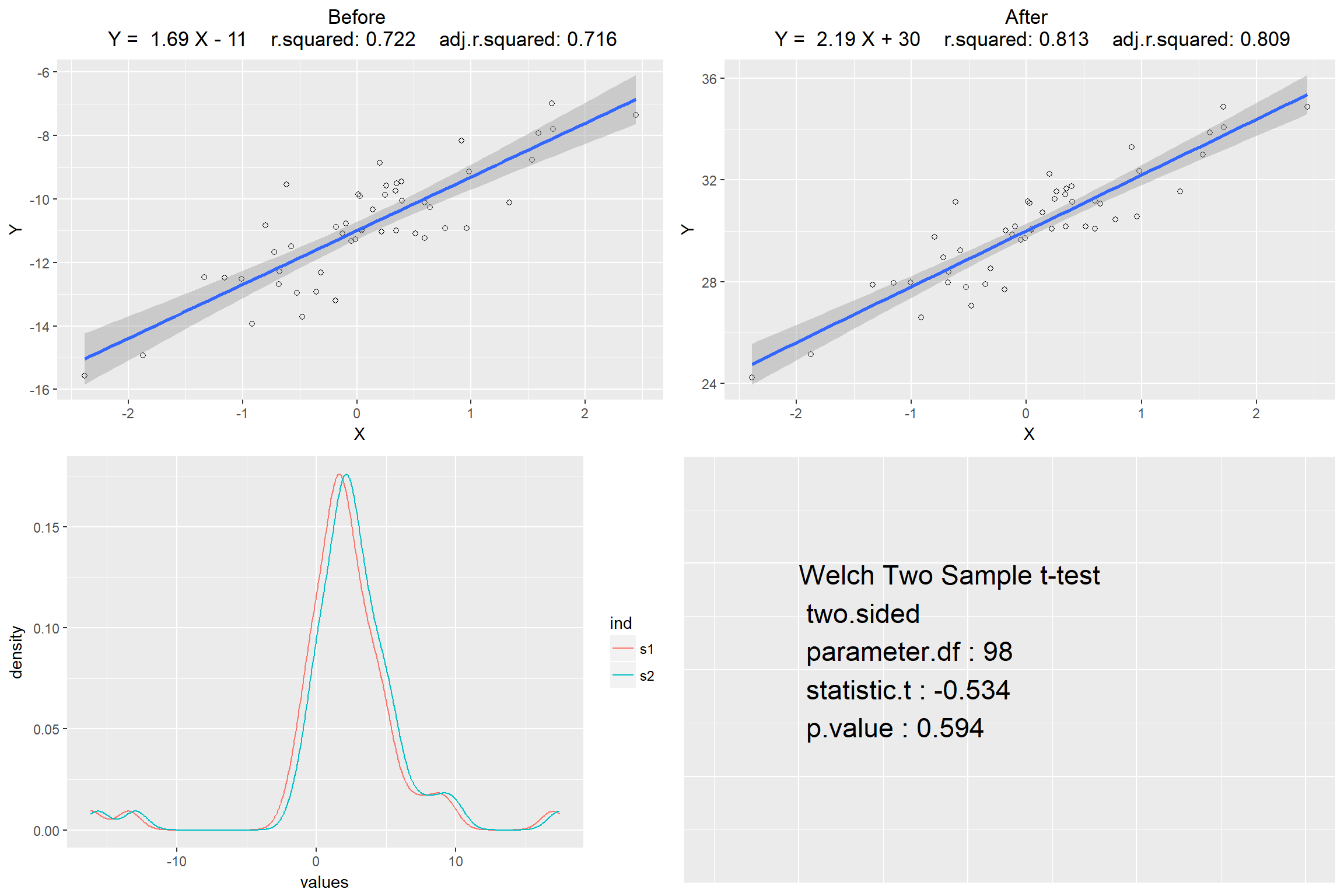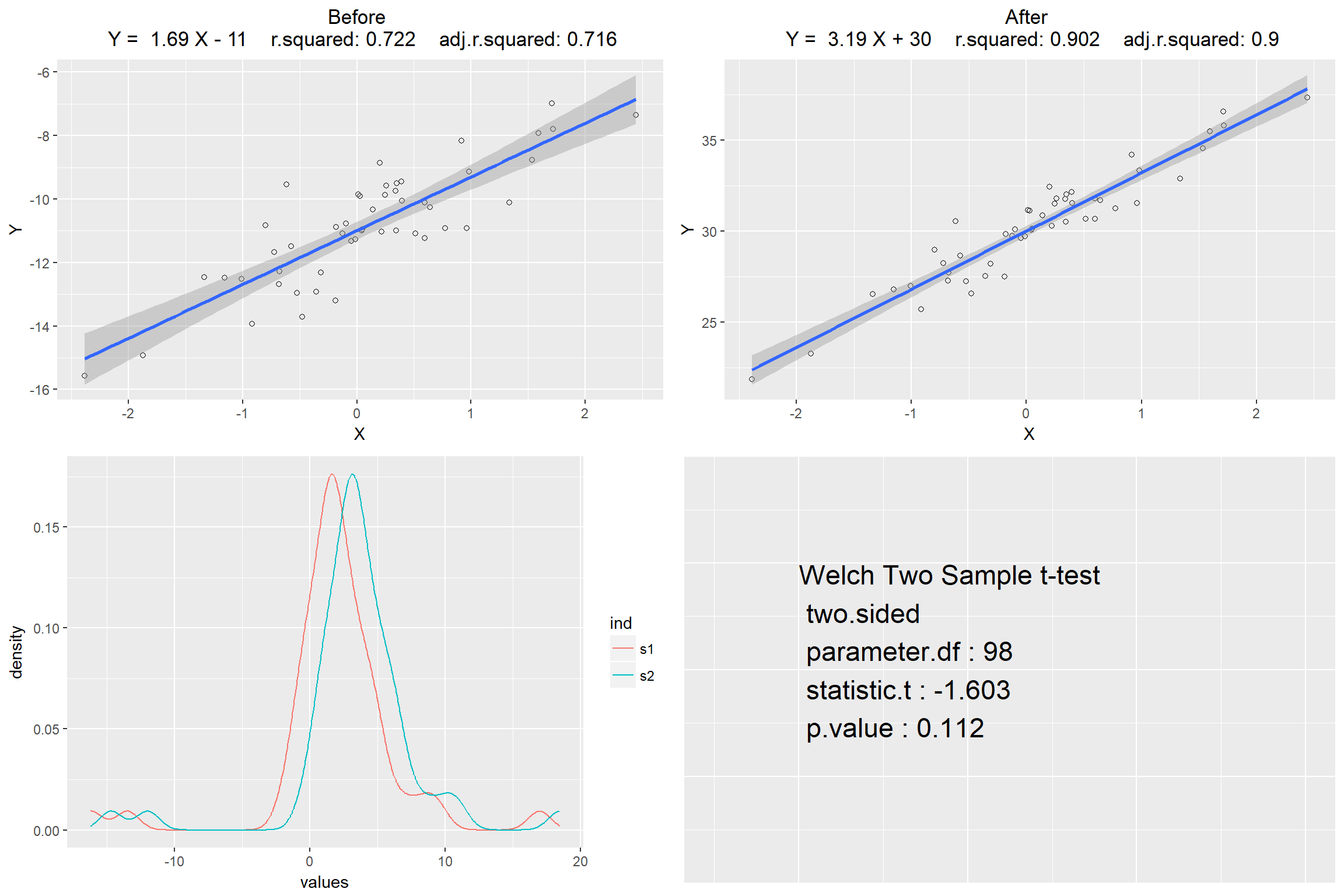### 1. Introduction

The purpose of this Article is to illustrate the method used in dependent sample hypothesis test which could contribute to analysing the variable relationship difference between before treatment and after the treatment. In other words, this method is used to test whether the treatment has significantly changed the relationship between sample variables.

### 2.The construct

This question was posed by Youshi on March 17, 2018. Imagine we have a group of subjects that having two variables, named Y and X. After we perform a control experiment by introducing a treatment we will have two samples, named samples-before and samples-after.The relevant information could be illustrated as follows.

##### samples-before
Dependent Variable Independent Variable
Yb1 Xb1
Yb2 Xb2
… … … …
Ybn Xbn
##### samples-after
Dependent Variable Independent Variable
Ya1 Xa1
Ya2 Xa2
… … … …
Yan Xan

First step is to perform regression analysis for two sample gourps sepreately and that would produce 4 scenarios.

##### Scenario 1
Dependent Variable Independent Variable Relationship
Yb Xb Strong Correlation
Ya Xa Strong Correlation
##### Scenario 2
Dependent Variable Independent Variable Relationship
Yb Xb Not Significant
Ya Xa Not Significant
##### Scenario 3
Dependent Variable Independent Variable Relationship
Yb Xb Not Significant
Ya Xa Strong Correlation
##### Scenario 4
Dependent Variable Independent Variable Relationship
Yb Xb Strong Correlation
Ya Xa Not Significant

It’s not hard to find that it is necessary to make a meaningful analysis further only under the scenario 1. For scenario 3 and 4, regression analysis already gives the answer that the relationship between dependent variable and independent variable are changed by treatment, from strong correlation to not significant correlation, or the oppsite way.

The next step is to design a statistic that could represent the relationship between variable Y and X. Assume variable Y and X are linear correlated.

$Y=\alpha·X+\beta\\$

$$\alpha$$ is slope and $$\beta$$ is interception. It’s expected that the mean of Y and X has the same linear correlation.

$\bar{Y}=\alpha·\bar{X}+\beta \\$

Then we’ll get… \begin{aligned} Y-\bar{Y} &=(\alpha·X + \beta) - (\alpha·\bar{X}+\beta) \\ &= \alpha(X-\bar{X})+(\beta-\beta) \\ &= \alpha(X-\bar{X}) \\ \end{aligned} So we get statistic $$\alpha$$ \begin{aligned} \alpha = \frac{Y-\bar{Y}}{X-\bar{X}} \end{aligned}

so we could use this linear transformation to get the statistic $$\alpha$$ of both sample before and sample after.

##### statistic $$\alpha$$
Before After
$$\alpha_{b1} = \frac{Y_{b1}-\bar{Y_{b}}}{X_{b1}-\bar{X_{b}}}$$ $$\alpha_{a1} = \frac{Y_{a1}-\bar{Y_{a}}}{X_{a1}-\bar{X_{a}}}$$
$$\alpha_{b2} = \frac{Y_{b2}-\bar{Y_{b}}}{X_{b2}-\bar{X_{b}}}$$ $$\alpha_{a2} = \frac{Y_{a2}-\bar{Y_{a}}}{X_{a2}-\bar{X_{a}}}$$
… … … …
$$\alpha_{bn} = \frac{Y_{bn}-\bar{Y_{b}}}{X_{bn}-\bar{X_{b}}}$$ $$\alpha_{an} = \frac{Y_{an}-\bar{Y_{a}}}{X_{an}-\bar{X_{a}}}$$

Caculate the sample mean, sample standard deviation and standard error.

$\bar{\alpha_{b}} = \frac{\sum\limits_{i=1}^{n}\alpha_{bi}}{n},\;\;\;\;\;\; \bar{\alpha_{a}} = \frac{\sum\limits_{i=1}^{n}\alpha_{ai}}{n},\;\;\;\;\;\; S_\alpha=\sqrt{\frac{\sum\limits_{i=1}^{n}(\alpha_{bi}-\alpha_{ai})^{2}}{n-1}},\;\;\;\;\;\; \sigma_\alpha=\frac{S}{\sqrt{n}}$

Caculate the t statistics.

\begin{aligned} t_\alpha=\frac{\bar{\alpha_b}-\bar{\alpha_a}}{\sigma} \end{aligned}

If $$t_\alpha$$ is significant, then we could reject null hypothesis and accept the alternative hypothesis that the treatment has significant influence on the relationship between Dependent Variable Y and Independent Variable X. But if $$t_\alpha$$ is not significant, we still need to test interception $$\beta$$ to give a further conclution.

\begin{aligned} &Y=\alpha·X+\beta\\ &\beta = Y - \alpha·X\\ \end{aligned}

Caculate the statistic $$\beta$$

##### statistic $$\beta$$
Before After
$$\beta_{b1} = {Y_{b1}-\alpha_{b}·X_{b1}}$$ $$\beta_{a1} = {Y_{a1}-\alpha_{a}·X_{a1}}$$
$$\beta_{b2} = {Y_{b2}-\alpha_{b}·X_{b2}}$$ $$\beta_{a1} = {Y_{a1}-\alpha_{a}·X_{a1}}$$
… … … …
$$\beta_{bn} = {Y_{bn}-\alpha_{b}·X_{bn}}$$ $$\beta_{an} = {Y_{an}-\alpha_{a}·X_{an}}$$

As previous test for $$\alpha$$, if $$t_\beta$$ is significant, then we could reject null hypothesis and accept the alternative hypothesis that the treatment has significant influence on the relationship between Dependent Variable Y and Independent Variable X. But if both $$t_\alpha$$ and $$t_\beta$$ are not significant, we then have a solid reason retain the null hypothesis.

### 3. Demos

First step is to produce two random data set x and C with each size = 50.

Secondly, construct a function named rmplot with 4 input parameters.They are slope, interception, residual coefficient, and the indicator for before treament of after treament. For example, rmplot(slope,interception,res,t). The out put is scattor polt.

Thirdly, build a function to perform t-test. The output is T-test result.

#### demo1

x1 <- rmplot(2,-11,1,0)
x2 <- rmplot(2.5,30,1,1)
ttestplot()#### demo2

x1 <- rmplot(2,-11,1,0)
x2 <- rmplot(3.5,30,1,1)
ttestplot()#### demo3

x1 <- rmplot(2,-11,1,0)
x2 <- rmplot(5,30,1,1)
ttestplot()